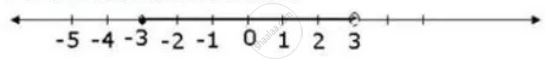Share

# Find the Range of Values of X Which Satisfies -2 2/3 <= X + 1/3 < 3 1/3; X in R Graph These Values of X on the Number Line. - Mathematics

Course

#### Question

Find the range of values of x which satisfies

-2 2/3 <= x + 1/3 < 3 1/3; x in R

Graph these values of x on the number line.

#### Solution

-2 2/3 <= x + 1/3 and x + 1/3 < 3  1/3

=> -8/3 <= x + 1/3 and x +1/3 < 10/3

=> -8/3 <= x + 1/3 and x +  1/3 < 10/3

=> -9/3 <=  x  and x < 9/3

=> -3 <= x and  x < 3

∴ -3 <= x < 3

The required graph of the solution set is:Is there an error in this question or solution?